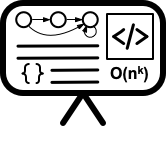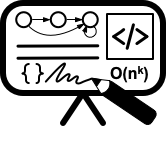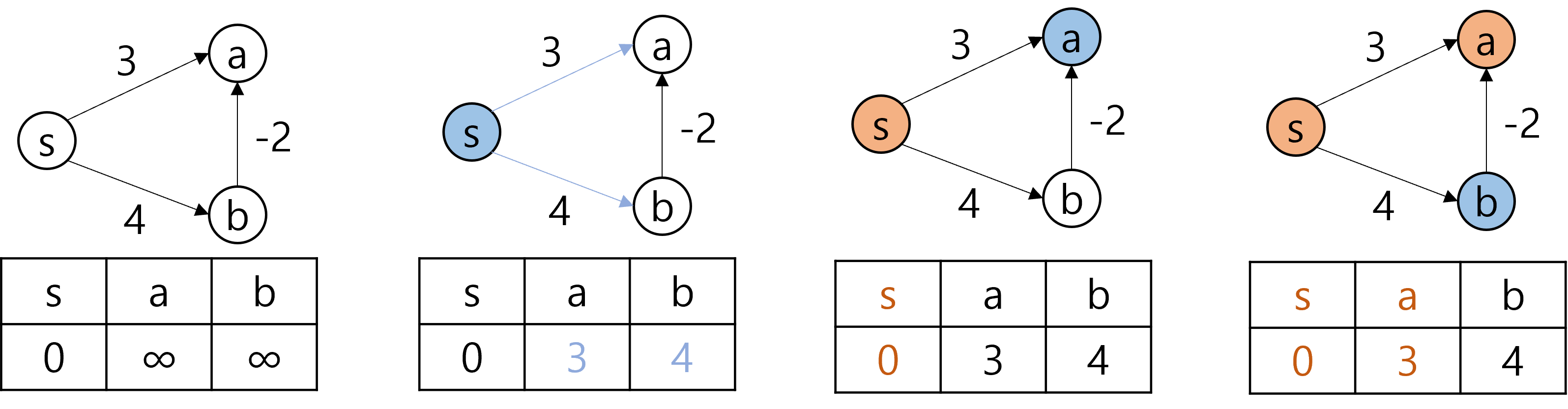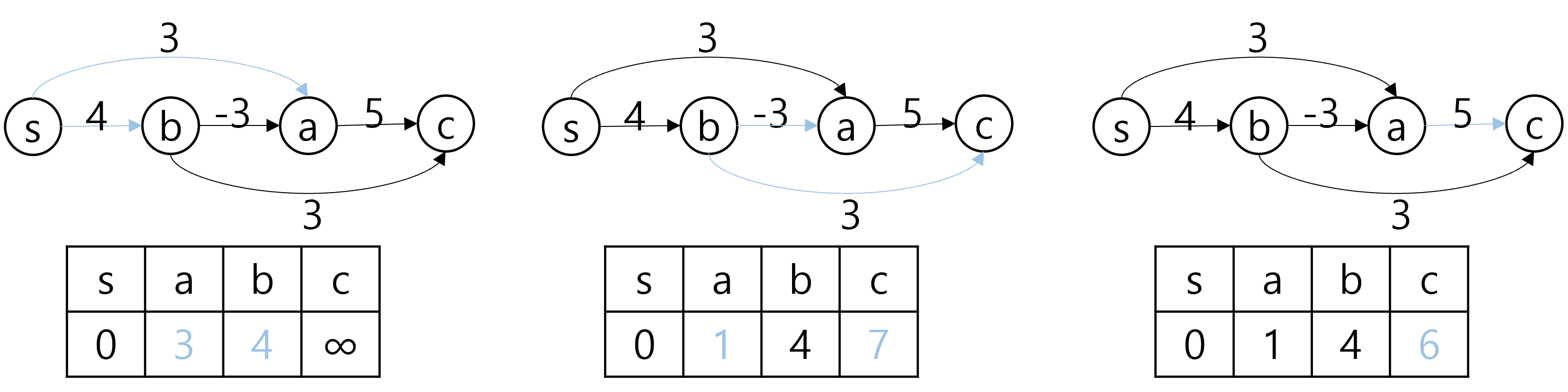# Lecture 17 - Shortest paths II - Bellman-Ford and Floyd-Warshall

Date Pre-lecture slides Post-lecture scribbles Lecture recording
October 24 2023## Notes

#### Dijkstra's Algorithm on Negative-weighted Graphs

As covered in the last lecture, Dijkstra’s algorithm finds the shortest distance from a single source vertex to all other vertices. However, when the graph contains edges with negative weights, Dijkstra’s algorithm might fail to find the shortest distance. Consider the following example.Suppose we start from the vertex $s$. Since the vertices $a$ and $b$ are both reachable from $s$, the distance to the two vertices would be updated to $3$ and $4$ respectively. Then we would visit vertex $a$, which is the closest unvisited vertex. There are no outgoing edges from $a$, so we do not update the distance at all. Lastly we visit vertex $b$. At this point, all other vertices are already marked as visited, so we do not update the distance table. Note that the actual shortest distance from $s$ to $a$ is $2$, which can be obtained by the path $s\rightarrow b \rightarrow a$. However, Dijkstra’s algorithm visits $a$ before it visits $b$ and mark $a$ as visited, which would result in incorrect shortest distance.

#### Bellman-Ford Algorithm

Bellman-Ford algorithm is an algorithm that finds the shortest path from a source vertex to all other vertices, like Dijkstra’s algorithm. The algorithm is slower compared to Dijkstra’s algorithm, but it can handle graphs with negative-weighted edges. At a high level, the algorithm runs as follows:

1. Initialize the distance to the source vertex as $0$, and the distance to all other vertices as $\infty$
2. For $|V|-1$ times: Check every edge $(u,v)$ and update distance if $d[v] < d[u]+l(u,v)$

Where $d[x]$ denotes the distance from the source vertex to the vertex $x$, and $l(u,v)$ denotes the length of the edge $(u,v)$. Since the algorithm loops for $|V|-1$ times, and each iteration involves checking every edge once, the runtime of the algorithm is $O(|V||E|)$.#### Properties of Bellman-Ford Algorithm

As mentioned in the previous section, Bellman-Ford algorithm can handle graphs with negative edges. This is because the algorithm scans over all edges on each iteration with excluding already visited vertices. Due to this behavior, Bellman-Ford Algorithm has a property that after $i$th iteration, the algorithm is guaranteed to discover the shortest distance to every other vertex that can be achieved by using at most $i$ edges. That is, the distances in the table $d$ after $i$th iteration is at least as good as the distance of the actual shortest path with at most $i$ edges.

One natural question we can ask is: Why do we iterate for $|V|-1$ times? How do we know that $|V|-1$ iterations are sufficient to find the shortest path? Suppose there is a path from the source $s$ to another vertex $v$ that uses $|V|$ edges. By pigeonhole principle, there exists a vertex $u$ that appears more than once in the path. In other words, this path definitely contains a cycle. Now suppose the cycle is a positive cycle(that is, the sum of the weights of the edges contained in the cycle is positive). In this case, taking the cycle can only increase the distance to $u$ and all the following vertices. Therefore, there is no point of considering a path containing the cycle when looking for the shortest distance. On the other hand, suppose the cycle is negative cycle. In this case, finding the shortest distance is not possible in the first place, since by repeatedly taking the cycle we can infinitely reduce the distance to certain vertices. Due to the above reasons, we are only interested in paths without cycles, which can only contain upto $|V|-1$ edges.

One last thing to note about Bellman-Ford algorithm is that it can be used to detect negative cycles. The idea comes from the properties of the algorithm described above. After $|V|-1$ iterations, Bellman-Ford algorithm finds the shortest distances to all vertices that can be achieved with $|V|-1$ edges. If the graph is free of negative cycles, then the distances in $d$ after $|V|-1$ iterations are the actual shortest distances that can possible be achieved. However, if the graph contains a negative cycle, then it would be possible to obtain shorter distance to certain vertices taking longer paths that contains the negative cycle. Therefore, by running $|V|$th iteration of Bellman-Ford algorithm and checking if the distance table is updated, we can check if the graph contains a negative cycle.

#### Single Source Shortest Distance on DAGs

If the graph does not contain a cycle, then we can find the shortest distance from a single source to all other vertices in linear time.

1. Topologically sort the graph
2. Initialize the distance to the source vertex as $0$, and the distance to all other vertices as $\infty$
3. For vertex $u\in V$ in topological order: Check every edge $(u,v)$ and update distance if $d[v]>d[u]+l(u,v)$

Once we have a topological sort of the graph, we know in which order the edges must be explored to get the shortest distance. Therefore, the DAG shortest distance algorithm only checks each edge once, which gives the overall time complexity of $O(|V|+|E|)$. Note that the algorithm can be applied on graphs with negative edges as long as the graph is acyclic.The operation of the algorithm illustrated in the figure seems similar to that of Bellman-Ford algorithm. However, Bellman-Ford algorithm had to iterate over all edges to find distances to update, whereas the DAG algorithm immediately knows which edges to check.

#### Floyd-Warshall Algorithm

Floyd-Warshall algorithm is another shortest distance algorithm for graphs with negative edges. However, instead of finding the distances from a single source, Floyd-Warshall algorithm finds the shortest distances between any pair of vertices. Floyd-Warshall algorithm recursively fills out a 3D array which would eventually hold the shortest distances. Suppose we labeled all vertices arbitrarily with integers $1,2,…,|V|$. Let $FW(i,j,k)$ denote the shortest distance from vertex $i$ to $j$ that can be achieved using only the intermediate vertices(excluding the source $i$ and the destination $j$) with labels less than or equal to $k$. As the base case, $FW(i,j,0)$ would equal to the length of the edge $(i,j)$ if the edge exists, and $\infty$ otherwise, since we cannot use any intermediate vertices. For $k>0$, there are two subcases to consider. If it is possible to obtain shorter distance by including the vertex $k$, then $FW(i,j,k)$ would equal to $FW(i,k,k-1)$+$FW(k,j,k-1)$, which is the sum of distances from $i$ to $k$ and from $k$ to $j$ using intermediate vertices upto $k-1$. On the other hand, if we can’t construct a shorter path by including $k$, then $FW(i,j,k)$ would be the same as $FW(i,j,k-1)$. Finally, $FW(i,j,|V|)$ would be the shortest distance between $i$ and $j$.

$FW(i,j,k)= \begin{cases} l(i,j) &\text{if }k=0 \text{ and }(i,j)\in E\\ \infty &\text{if }n=0 \text{ and }(i,j)\notin E\\ \min(FW(i,j,k-1),FW(i,k,k-1)+FW(k,j,k-1)) &\text{otherwise} \end{cases}$

Filling out the 3D array takes $O(|V|^3)$.

• Textbooks
• Erickson, Jeff. Algorithms
• Skiena, Steven. The Algorithms Design Manual
• Chapter 8.3 - Shortest Path
• Sedgewick, Robert and Wayne, Kevin. Algorithms (Forth Edition)
• Chapter 4.4 - Shortest Paths
• Cormen, Thomas, et al. Algorithms (Forth Edition)
• Chapter 22 - Single Source Shortest Path
• Chapter 23 - All-Pairs Shortest Paths
• Sariel’s Lecture 18